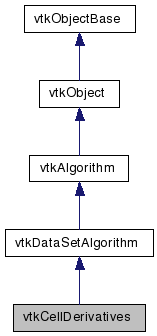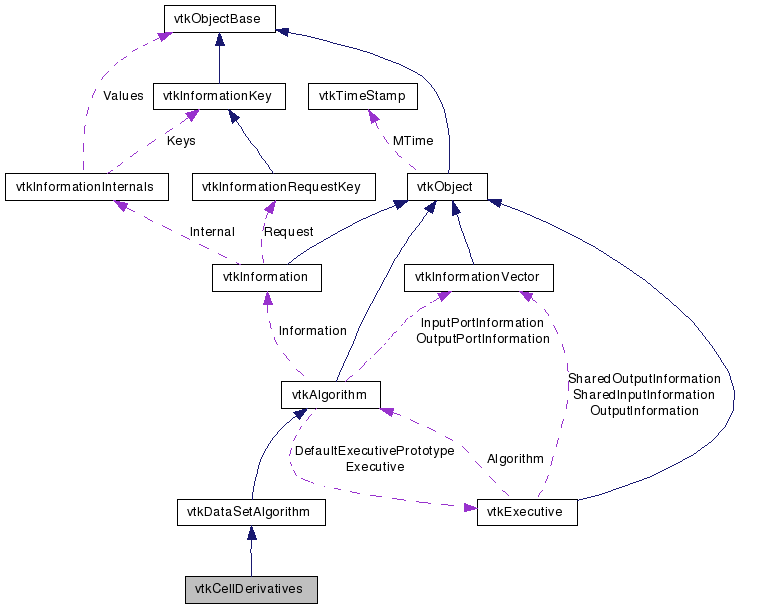# vtkCellDerivatives Class Reference

`#include <vtkCellDerivatives.h>`

Inheritance diagram for vtkCellDerivatives:[legend]
Collaboration diagram for vtkCellDerivatives:[legend]

## Detailed Description

compute derivatives of scalars and vectors

vtkCellDerivatives is a filter that computes derivatives of scalars and vectors at the center of cells. You can choose to generate different output including the scalar gradient (a vector), computed tensor vorticity (a vector), gradient of input vectors (a tensor), and strain matrix of the input vectors (a tensor); or you may choose to pass data through to the output.

Note that it is assumed that on input scalars and vector point data is available, which are then used to generate cell vectors and tensors. (The interpolation functions of the cells are used to compute the derivatives which is why point data is required.)

Warning:
The computed derivatives are cell attribute data; you can convert them to point attribute data by using the vtkCellDataToPointData filter. Note that, due to the interpolation function used (obtained using 1/r**2 normalized sum), the derivatives calculated for polygons with more than 4 vertices are inaccurate in most cases.

The point data is passed through the filter to the output.

vtkVectorNorm
Tests:
vtkCellDerivatives (Tests)

Definition at line 60 of file vtkCellDerivatives.h.

## Public Types

typedef vtkDataSetAlgorithm Superclass

## Public Member Functions

virtual const char * GetClassName ()
virtual int IsA (const char *type)
void PrintSelf (ostream &os, vtkIndent indent)
virtual void SetVectorMode (int)
virtual int GetVectorMode ()
void SetVectorModeToPassVectors ()
void SetVectorModeToComputeVorticity ()
const char * GetVectorModeAsString ()
virtual void SetTensorMode (int)
virtual int GetTensorMode ()
void SetTensorModeToPassTensors ()
void SetTensorModeToComputeStrain ()
const char * GetTensorModeAsString ()

## Static Public Member Functions

static int IsTypeOf (const char *type)
static vtkCellDerivativesSafeDownCast (vtkObject *o)
static vtkCellDerivativesNew ()

## Protected Member Functions

vtkCellDerivatives ()
~vtkCellDerivatives ()
int RequestData (vtkInformation *, vtkInformationVector **, vtkInformationVector *)

int VectorMode
int TensorMode

## Member Typedef Documentation

Reimplemented from vtkDataSetAlgorithm.

Definition at line 63 of file vtkCellDerivatives.h.

## Constructor & Destructor Documentation

 vtkCellDerivatives::vtkCellDerivatives ( ) ` [protected]`

 vtkCellDerivatives::~vtkCellDerivatives ( ) ` [inline, protected]`

Definition at line 105 of file vtkCellDerivatives.h.

## Member Function Documentation

 virtual const char* vtkCellDerivatives::GetClassName ( ) ` [virtual]`

Reimplemented from vtkDataSetAlgorithm.

 static int vtkCellDerivatives::IsTypeOf ( const char * name ) ` [static]`

Return 1 if this class type is the same type of (or a subclass of) the named class. Returns 0 otherwise. This method works in combination with vtkTypeRevisionMacro found in vtkSetGet.h.

Reimplemented from vtkDataSetAlgorithm.

 virtual int vtkCellDerivatives::IsA ( const char * name ) ` [virtual]`

Return 1 if this class is the same type of (or a subclass of) the named class. Returns 0 otherwise. This method works in combination with vtkTypeRevisionMacro found in vtkSetGet.h.

Reimplemented from vtkDataSetAlgorithm.

 static vtkCellDerivatives* vtkCellDerivatives::SafeDownCast ( vtkObject * o ) ` [static]`

Reimplemented from vtkDataSetAlgorithm.

 void vtkCellDerivatives::PrintSelf ( ostream & os, vtkIndent indent ) ` [virtual]`

Methods invoked by print to print information about the object including superclasses. Typically not called by the user (use Print() instead) but used in the hierarchical print process to combine the output of several classes.

Reimplemented from vtkDataSetAlgorithm.

 static vtkCellDerivatives* vtkCellDerivatives::New ( ) ` [static]`

Construct to compute the gradient of the scalars and vectors.

Reimplemented from vtkDataSetAlgorithm.

 virtual void vtkCellDerivatives::SetVectorMode ( int ) ` [virtual]`

Control how the filter works to generate vector cell data. You can choose to pass the input cell vectors, compute the gradient of the input scalars, or extract the vorticity of the computed vector gradient tensor. By default (VectorModeToComputeGradient), the filter will take the gradient of the input scalar data.

 virtual int vtkCellDerivatives::GetVectorMode ( ) ` [virtual]`

Control how the filter works to generate vector cell data. You can choose to pass the input cell vectors, compute the gradient of the input scalars, or extract the vorticity of the computed vector gradient tensor. By default (VectorModeToComputeGradient), the filter will take the gradient of the input scalar data.

 void vtkCellDerivatives::SetVectorModeToPassVectors ( ) ` [inline]`

Control how the filter works to generate vector cell data. You can choose to pass the input cell vectors, compute the gradient of the input scalars, or extract the vorticity of the computed vector gradient tensor. By default (VectorModeToComputeGradient), the filter will take the gradient of the input scalar data.

Definition at line 77 of file vtkCellDerivatives.h.

 void vtkCellDerivatives::SetVectorModeToComputeGradient ( ) ` [inline]`

Control how the filter works to generate vector cell data. You can choose to pass the input cell vectors, compute the gradient of the input scalars, or extract the vorticity of the computed vector gradient tensor. By default (VectorModeToComputeGradient), the filter will take the gradient of the input scalar data.

Definition at line 79 of file vtkCellDerivatives.h.

 void vtkCellDerivatives::SetVectorModeToComputeVorticity ( ) ` [inline]`

Control how the filter works to generate vector cell data. You can choose to pass the input cell vectors, compute the gradient of the input scalars, or extract the vorticity of the computed vector gradient tensor. By default (VectorModeToComputeGradient), the filter will take the gradient of the input scalar data.

Definition at line 81 of file vtkCellDerivatives.h.

 const char* vtkCellDerivatives::GetVectorModeAsString ( )

Control how the filter works to generate vector cell data. You can choose to pass the input cell vectors, compute the gradient of the input scalars, or extract the vorticity of the computed vector gradient tensor. By default (VectorModeToComputeGradient), the filter will take the gradient of the input scalar data.

 virtual void vtkCellDerivatives::SetTensorMode ( int ) ` [virtual]`

Control how the filter works to generate tensor cell data. You can choose to pass the input cell tensors, compute the gradient of the input vectors, or compute the strain tensor of the vector gradient tensor. By default (TensorModeToComputeGradient), the filter will take the gradient of the vector data to construct a tensor.

 virtual int vtkCellDerivatives::GetTensorMode ( ) ` [virtual]`

Control how the filter works to generate tensor cell data. You can choose to pass the input cell tensors, compute the gradient of the input vectors, or compute the strain tensor of the vector gradient tensor. By default (TensorModeToComputeGradient), the filter will take the gradient of the vector data to construct a tensor.

 void vtkCellDerivatives::SetTensorModeToPassTensors ( ) ` [inline]`

Control how the filter works to generate tensor cell data. You can choose to pass the input cell tensors, compute the gradient of the input vectors, or compute the strain tensor of the vector gradient tensor. By default (TensorModeToComputeGradient), the filter will take the gradient of the vector data to construct a tensor.

Definition at line 94 of file vtkCellDerivatives.h.

 void vtkCellDerivatives::SetTensorModeToComputeGradient ( ) ` [inline]`

Control how the filter works to generate tensor cell data. You can choose to pass the input cell tensors, compute the gradient of the input vectors, or compute the strain tensor of the vector gradient tensor. By default (TensorModeToComputeGradient), the filter will take the gradient of the vector data to construct a tensor.

Definition at line 96 of file vtkCellDerivatives.h.

 void vtkCellDerivatives::SetTensorModeToComputeStrain ( ) ` [inline]`

Control how the filter works to generate tensor cell data. You can choose to pass the input cell tensors, compute the gradient of the input vectors, or compute the strain tensor of the vector gradient tensor. By default (TensorModeToComputeGradient), the filter will take the gradient of the vector data to construct a tensor.

Definition at line 98 of file vtkCellDerivatives.h.

 const char* vtkCellDerivatives::GetTensorModeAsString ( )

Control how the filter works to generate tensor cell data. You can choose to pass the input cell tensors, compute the gradient of the input vectors, or compute the strain tensor of the vector gradient tensor. By default (TensorModeToComputeGradient), the filter will take the gradient of the vector data to construct a tensor.

 int vtkCellDerivatives::RequestData ( vtkInformation * , vtkInformationVector ** , vtkInformationVector * ) ` [protected, virtual]`

This is called within ProcessRequest when a request asks the algorithm to do its work. This is the method you should override to do whatever the algorithm is designed to do. This happens during the fourth pass in the pipeline execution process.

Reimplemented from vtkDataSetAlgorithm.

## Member Data Documentation

 int vtkCellDerivatives::VectorMode` [protected]`

Definition at line 108 of file vtkCellDerivatives.h.

 int vtkCellDerivatives::TensorMode` [protected]`

Definition at line 109 of file vtkCellDerivatives.h.

The documentation for this class was generated from the following file:

Generated on Wed Jun 3 18:56:12 2009 for VTK by1.5.6